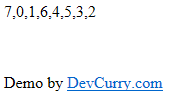# Array Shuffle in JavaScript

 Tweet
I have yet to find a script that truly does a random shuffle across all browsers using JavaScript (no frameworks). However there are a couple of scripts I use, that are ‘good-enough’ to be used when an Array has to be shuffled. One of them which works well with a large set of records and handles good amount of iterations without hanging the browser is the Microsoft’s Shuffle alogrithm. I learnt about this algorithm from a blog post by RobWeir

The script is as follows:

```<script type="text/javascript" language="javascript">
function ArrayShuffle(a) {
var d,
c,
b = a.length;
while (b) {
c = Math.floor(Math.random() * b);
d = a[--b];
a[b] = a[c];
a[c] = d
}
return a;
}

var lst = [0, 1, 2, 3, 4, 5, 6, 7];
document.write(ArrayShuffle(lst));
</script>
```

The code above works well, atleast for my requirements of shuffling an array 2000 times every hour. However you need to evaluate your context and make changes in the script, if needed.Tip: Increase the array length (atleast 7 and above) if you want to test randomness.